Five resistors are connected as shown in the diagram. The equivalent resistance between A and B is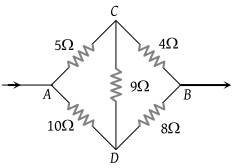(1) 6 ohm

(2) 9 ohm

(3) 12 ohm

(4) 15 ohm

Concept Questions :-

Wheatstone bridge
High Yielding Test Series + Question Bank - NEET 2020

Difficulty Level:

In the figure given the value of X resistance will be, when the p.d. between B and D is zero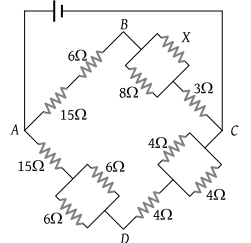(1) 4 ohm

(2) 6 ohm

(3) 8 ohm

(4) 9 ohm

Concept Questions :-

Wheatstone bridge
High Yielding Test Series + Question Bank - NEET 2020

Difficulty Level:

The effective resistance between points A and B is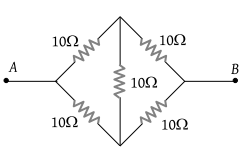(1) 10 Ω

(2) 20 Ω

(3) 40 Ω

(4) None of the above three values

Concept Questions :-

Wheatstone bridge
High Yielding Test Series + Question Bank - NEET 2020

Difficulty Level:

Five resistors of given values are connected together as shown in the figure. The current in the arm BD will be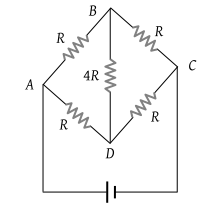(1) Half the current in the arm ABC

(2) Zero

(3) Twice the current in the arm ABC

(4) Four times the current in the arm ABC

Concept Questions :-

Wheatstone bridge
High Yielding Test Series + Question Bank - NEET 2020

Difficulty Level:

In the network shown in the figure, each of the resistance is equal to 2 Ω. The resistance between the points A and B is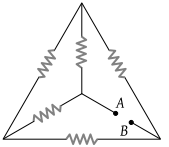(1) 1 Ω

(2) 4 Ω

(3) 3 Ω

(4) 2 Ω

Concept Questions :-

Wheatstone bridge
High Yielding Test Series + Question Bank - NEET 2020

Difficulty Level:

In the arrangement of resistances shown below, the effective resistance between points A and B is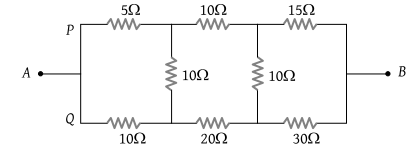(1) 20 Ω

(2) 30 Ω

(3) 90 Ω

(4) 110 Ω

Concept Questions :-

Wheatstone bridge
High Yielding Test Series + Question Bank - NEET 2020

Difficulty Level:

Five resistances are connected as shown in the figure. The effective resistance between the points A and B is :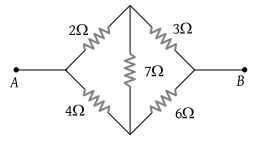(1) $\frac{10}{3}\Omega$

(2) $\frac{20}{3}\Omega$

(3) 15 Ω

(4) 6 Ω

Concept Questions :-

Wheatstone bridge
High Yielding Test Series + Question Bank - NEET 2020

Difficulty Level:

In the given figure, when galvanometer shows no deflection, the current (in ampere) flowing through 5 Ω resistance will be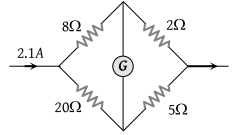(1) 0.5

(2) 0.6

(3) 0.9

(4) 1.5

Concept Questions :-

Wheatstone bridge
High Yielding Test Series + Question Bank - NEET 2020

Difficulty Level:

In the Wheatstone's bridge shown, P = 2 Ω, Q = 3 Ω, R = 6 Ω and S = 8 Ω. In order to obtain balance, shunt resistance across 'S' must be [SCRA 1998]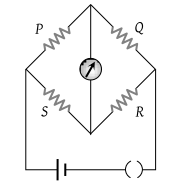(1) 2 Ω

(2) 3 Ω

(3) 6 Ω

(4) 8 Ω

Concept Questions :-

Wheatstone bridge
High Yielding Test Series + Question Bank - NEET 2020

Difficulty Level:

Potential difference between the points P and Q in the electric circuit shown is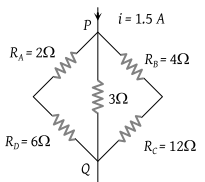(1) 4.5 V

(2) 1.2 V

(3) 2.4 V

(4) 2.88 V

Concept Questions :-

Combination of resistors